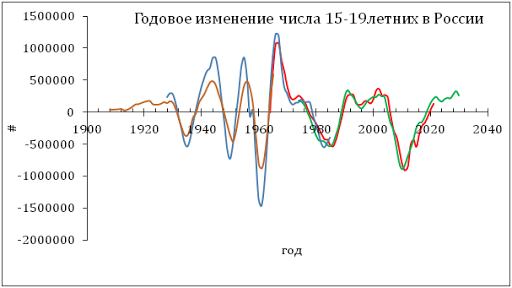## 8/13/13

### Long term problems of the labor force market

A month ago we presented a prediction of the labor force participation rate, LFPR, measured by the Bureau of Labor Statistics. The LFPR (the portion of people in labor force) for the working age population (16 years of age and over) has been on a long-term decline since 1995. We predicted the fall down to 59% by 2025. Here we use a different approximation to project the future evolution of the LFPR. Following the Kondratiev wave approach (the Russian economist Kondratiev introduced long-period (50 to 60 years) waves in economic evolution – see Figure 1) we interpolated the observed LFPR curve by a sinus function with a period of ~70 years. The result is shown in Figure 2. The trough of the model function is 2030 and the bottom rate in 58.5%.

Another interesting feature is shown in Figure 3. The rate of unemployment also has some long period (~35 years)  oscillation in amplitude together with short-period ( 7 to 11 years) fluctuations. The period of 35 years is a half of the labor force period. The rate of unemployment is sensitive to the peaks and inflection points of the labor force curve. One may expect the following unemployment peak to be higher than in 2010.

Figure 1. The Kondratiev wave

Figure 2. The actual LFPR curve (red) and that predicted by sinus function with a period of ~70 years.

Figure 3.  The rate of unemployment.